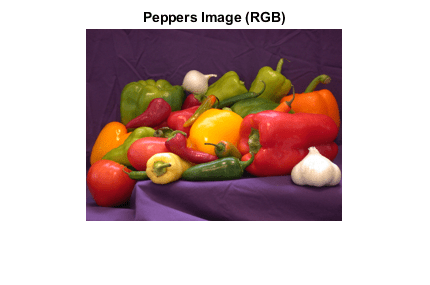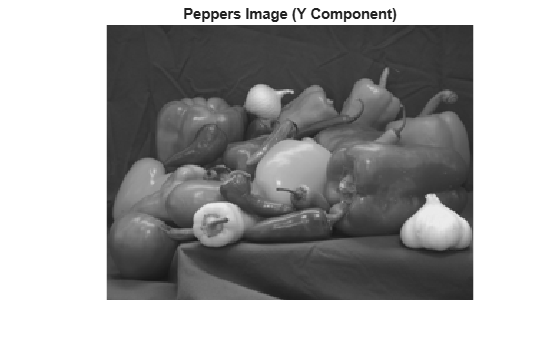# write

## 语法

``write(t,imageData)``
``write(t,Y,Cb,Cr)``

## 说明

``write(t,imageData)` 将 `imageData` 写入到与 `Tiff` 对象 `t` 关联的 TIFF 文件中。`write` 方法将数据划分为条带或图块，具体取决于 `RowsPerStrip` 标记或 `TileLength` 和 `TileWidth` 标记的值。`

``write(t,Y,Cb,Cr)` 将 YCbCr 分量数据写入到 TIFF 文件中。仅为拥有 YCbCr 光度表示的图形使用此语法。`

## 示例

```load('peppers_RGB.mat'); imshow(RGB); title('Peppers Image (RGB)');````t = Tiff('myfile.tif','w'); `

```tagstruct.ImageLength = size(RGB,1); tagstruct.ImageWidth = size(RGB,2); tagstruct.Photometric = Tiff.Photometric.RGB; tagstruct.BitsPerSample = 8; tagstruct.SamplesPerPixel = 3; tagstruct.PlanarConfiguration = Tiff.PlanarConfiguration.Chunky; tagstruct.Software = 'MATLAB'; ```

`setTag(t,tagstruct)`

```write(t,RGB); close(t);```

```load('peppers_YCbCr.mat'); imshow(Y); title('Peppers Image (Y Component)');````t = Tiff('myfile_YCbCr.tif','w'); `

```tagstruct.ImageLength = size(Y,1); tagstruct.ImageWidth = size(Y,2); tagstruct.SampleFormat = 1; % uint tagstruct.Photometric = Tiff.Photometric.YCbCr; tagstruct.BitsPerSample = 8 ; tagstruct.SamplesPerPixel = 3; tagstruct.YCbCrSubSampling = [1,1]; tagstruct.Compression = Tiff.Compression.None; tagstruct.PlanarConfiguration = Tiff.PlanarConfiguration.Chunky; tagstruct.Software = 'MATLAB'; ```

`setTag(t,tagstruct)`

```write(t,Y,Cb,Cr) close(t)```

## 提示

• 不建议写那些具有某些光度配置和每像素采样数的 TIFF 图像。`SamplesPerPixel` 的值必须等于 `Tiff` 对象中指定的 `Photometric` 颜色通道数和 `ExtraSamples` 的总和。